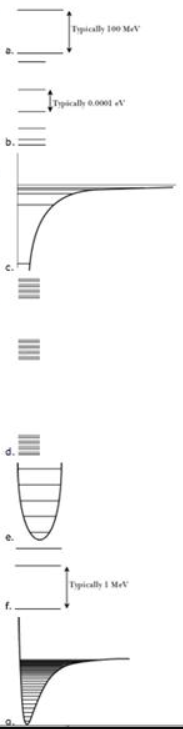# Problem: Match the type of system or situation to the appropriate energy level diagram. idealized quantized spring-mass oscillator

###### FREE Expert Solution

The energy of quantum harmonic oscillator:

$\overline{){{\mathbf{E}}}_{{\mathbf{n}}}{\mathbf{=}}{\mathbf{\left(}}{\mathbf{n}}{\mathbf{+}}\frac{\mathbf{1}}{\mathbf{2}}{\mathbf{\right)}}{\mathbf{\hslash }}{\mathbf{\omega }}}$

ℏ = h/(2π), n is the energy level, n = 0, 1, 2, 3...

88% (205 ratings)###### Problem Details

Match the type of system or situation to the appropriate energy level diagram.

idealized quantized spring-mass oscillator